2009-04-27 Fun with quantifiers (en)

Some time ago, a friend of mine asked me how to put a symbol below a quantifier—in other words, how to typeset e.g.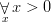instead of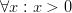(this is quite common in Polish typography).

That’s easy, I said. You just put \limits right after the \forall. Well, easy as it was, it didn’t work. The reason is obvious (at least, after a reading session with The TeXbook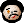): \limits (and it’s twin, \nolimits) work only after so-called operators. For example, you can get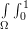when you say

\int\limits_\Omega \int\nolimits_0^1

to (La)TeX. (Note that LaTeX, and particularly AMS-LaTeX, has some defaults, different for, e.g., sums and integrals. Note also that you may change them globally: either by using some options of AMS-LaTeX, or by saying something like

\let\originalint=\int
\renewcommand{\int}{\originalint\limits}

in the preamble of the LaTeX file; plain TeX or ConTeXt users would use \def\int{...} instead of \newcommand{\int}{...}.)

Since by default, \forall is an atom of type Ord (as in “Ordinary symbol”; for discussion of atoms and their types, see Chapter 17 of The TeXbook), it can’t take \limits (neither \nolimits). It is easy, however, to convince TeX to treat it as an Op (”Operator”) atom: you just have to say \mathop\forall instead of just \forall. This way, you may type

$\mathop\forall\limits_x x>0$

to get the result shown above.

Note that this has also an interesting side-effect: Op atoms, unlike Ord ones, are vertically centered. I don’t think this is a problem, but if you really don’t like it, here’s how to avoid it:

$\mathop{\hbox{$\forall$}}\limits_x x>0$;

don’t ask me why this works, just look at Rule 13 of Appendix G in The TeXbook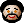.

But this is not the end of the story. The quantifier symbol, even with \mathop, does not really behave like an operator: it has a fixed, rather small, size; we would sometimes like to have it a bit bigger. (In fact, it would be best if its size adapted to different circumstances—but I guess it can be safely assumed that no-one puts quantifiers into sub- or superscripts, so I don’t want to go into this kind of details. If you really want to know how to do this, look up \mathchoice in The TeXbook‘s index.) An easy solution would be to say

$\mathop{\large\forall}\limits_x x>0$

or something similar; again, this does not work. The reason (sorry to say that again, but this is explained in detail… well, you know where) is that TeX chooses fonts for a math formula on the basis of current settings at the end of the formula; maybe because of that, if you try to use \large in a math formula, you get a LaTeX Font Warning: Command \large invalid in math mode...

This way, we have at least two choices. One is to redefine some (La)TeX internals and start to mess up with font families, mathcodes etc. I didn’t want to do this, since the thing is complicated even in plain TeX. The easier solution might be to try the \hbox trick again (notice the two occurences of \mathop):

$\mathop{\hbox{\Large$\mathop\forall$}}\limits_x x>0$,

which yields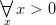. However, this is again not very good—the “for all” symbol seems shifted a bit up. This is true: although it is centered around the so-called vertical axis, the axis height is taken from the \Large size, i.e., it is higher than it should be here. How to overcome this new problem? Again, it is (of course!) possible, and in fact not that difficult: you just write

$\mathop{\vcenter{\hbox{\Large$\forall$}}}\limits_x x>0$

and finally get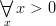! (Note that the \hbox is necessary here, since \vcenter takes vertical material as its argument; try removing the \hbox and see (and explain) what happens! Also, the second \mathop is now made obsolete by \vcenter.)

Obviously, if you need such a construct, it is best to use a macro so that you don’t have to repeat the above code every time you need a quantifier; for example, in LaTeX you write

\let\originalforall=\forall
\renewcommand{\forall}{\mathop{\vcenter{\hbox{\Large$\originalforall$}}}}

and use \forall as usual (with or even without subscripts).

Two final remarks are:

1. Yes, I know that you want to say that the example formula is wrong. It isn’t; I just forgot to mention that it should be interpreted in the universe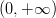.
2. Even the last thing is not really (typographically) optimal: the best solution would be to use a font with a predesigned large “for all” symbol (which is absent from Computer Modern fonts; also the Comprehensive LaTeX Symbol List does not mention any font containing it…). The used way (with \Large and similar commands) may result in incosistent line widths etc. In case of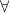, this seems to be tolerable: you get thicker lines, which make the symbol visually heavier, but still the lines are thinner than in, e.g.,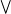(\bigvee). But I’m not a font designer, and thus can’t help this problem…